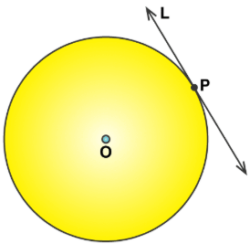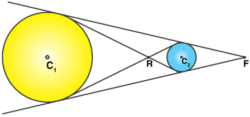Win up to 100% scholarship on Aakash BYJU'S JEE/NEET courses with ABNAT Win up to 100% scholarship on Aakash BYJU'S JEE/NEET courses with ABNAT

# Common Tangents in Coordinate Geometry

A line touching the circle at a point can be called a tangent to a circle. At the tangency point, the radius is always perpendicular to the tangent of a circle.

The following are the properties of tangents:

• A tangent will never cross the circle but touches it.
• An angle is formed by the chord and tangent, which is the same as the angle that is being inscribed on the opposite side of the chord.
• The tangents of a circle are equal if it is emerging from the same external point.## What Are Common Tangents in Coordinate Geometry?

A line which is tangent to more than one circle is called a common tangent. The tangents can be classified into common tangents that are internal and external. An internal tangent is a line segment, which passes through the centre of the two circles, whereas the external common tangents do not.

Direct Common Tangents

It is the tangents dividing externally in the ratio of the radii and meeting on the line of centres.

Transverse Common Tangents

It is the tangents dividing internally in the ratio of the radii and meeting on the line of centres.## Common Tangents in Coordinate Geometry Condition and Formulae

1. Number of Tangents Condition
• 4 common tangents r1 + r2 < c1c2
• 3 common tangents r1 + r2 = c1c2
• 2 common tangents |r1 – r2| < c1c2 < r1 + r2
• 1 common tangent |r1 – r2| = c1c2
• no common tangents c1c2 < |r1 – r2|

The formula for the tangents of the two circles with respective centres and radii.

(i) Internally: If |C1 C2| = |r2 – r1| and the point of contact is ((r1 x2 – r2 x1)/(r1 + r2) , (r1y2 – r2y1)/(r1 + r2)).

(ii) Externally: If |C1 C2| = |r2 – r1| and the point of contact is ((r1 x2 + r2 x1)/(r1 + r2) , (r1y2 + r2y1)/(r1 + r2)).

3D Geometry

Tangents and Normals

## Solved Problems on Common Tangents

Example 1: What is the angle between the two tangents from the origin to the circle (x − 7)2 + (y + 1)2 = 25?

Solution:

Any line through (0, 0) be y − mx = 0, and it is a tangent to circle (x − 7)2 + (y + 1)2 = 25, if [−1 − 7m] / √[1 + m2] = 5

⇒ m = 3 / 4, −4 / 3

Therefore, the product of both slopes is -1

i.e., [3 / 4]* [−4 / 3] = −1

Hence, the angle between the two tangents is π/2.

Example 2: A pair of tangents are drawn from the origin to the circle x2 + y2 + 20 (x + y) + 20 = 0. Find the equation of the pair of tangents.

Solution:

The equation of pair of tangents is given by SS1 = T2.

Here, S = x2 + y2 + 20 (x + y) + 20, S1 = 20

T = 10 (x + y) + 20

∴ SS1 = T2

⇒20 {x2 + y2 + 20 (x + y) + 20} = 102 (x + y+ 2)2

⇒4x2 + 4y2 + 10xy = 0

⇒2x2 + 2y2 + 5xy = 0

Example 3: What are the equations of the tangents to the circle x2 + y2 −6x + 4y = 12, which are parallel to the straight line 4x + 3y + 5 = 0?

Solution:

Let equation of tangent be 4x + 3y + 5 = 0, then √[9 + 4 + 12] =∣[4(3) + 3(−2) + k] / √[16+9]∣

⇒ 6 + k = ±25

⇒ k = 19 and −31

Hence, the tangents are 4x + 3y + 19 = 0 and 4x + 3y −31 = 0.

Example 4: Calculate the area of the triangle formed by the tangents from the points (h, k) to the circle x2 + y2 = a2 and the line joining their points of contact.

Solution:

Equation of chord of contact AB is xh + yk = a2 …..(i)

OM = Length of the perpendicular from O (0, 0) on line (i) = a2 / √[h2 + k2]

∴ AB = 2AM = 2 √OA2 − OM2

= 2a √[h2 + k2−a2] / √[h2 + k2]

Also, PM = Length of perpendicular from P(h, k) to the line (i) is [h2 + k2−a2] / √[h2 + k2]. Therefore, the required area of triangle PAB = [1 / 2] * AB * PM = [a (h2 + k2−a2)3/2] / [h2 + k2].

Example 5: Find the number of common tangents to the circles x2 + y2 − 4x − 6y − 12 = 0 and x2 + y2 + 6x + 18y + 26 = 0.

Solution:

Centres of circles are C1 (2, 3) and C2 (−3, −9), and their radii are r1 = 5 and r2 = 8. Obviously, r1 + r2 = C1C2, i.e., circles touch each other externally.

Hence, there are three common tangents.

Example 6: What is the gradient of the tangent line at the point (acosα, asinα) to the circle x2 + y2 = a2?

Solution:

The equation of a tangent at (a cos α, a sin α) to the circle x2 + y2 = a2 is a x cos α + a y sin α = a2.

Hence, its gradient is [−a cos α] / [a sin α] = − cot α.

Example 7: Find the number of common tangents to the circles x2 + y2 + 2x + 8y – 23 = 0 and x2 + y2 – 4x – 10y – 19 = 0 is

Solution:

Let

x2 + y2 + 2x + 8y – 23 = 0….(1)

And x2 + y2 – 4x – 10y – 19 = 0 …..(2)

The centre of the circle is given by C (-g, -f).

The centre of the circle (1) is (-1, -4), and of the circle (2) is (2, 5).

The radius of the circle is given by

$$\begin{array}{l}r=\sqrt{(g^{2}+f^{2}-c)}\\ r_{1}=\sqrt{(1^2+16+23)}\\ r_{1}=\sqrt{40}\\r_{2}=\sqrt{(2^{2}+5^{2}+19)}\\ r_{2}=\sqrt{48}\\\end{array}$$

$$\begin{array}{l}r_{1}+r_{2}>C_{1}C_{2}\\ |r_{1}-r_{2}|<C_{1}C_{2}\\\end{array}$$

The above two conditions are obtained.

There exist 3 scenarios.

• Two circles intersect each other.
• Two circles just touch the outer surface of each other.
• Two circles don’t touch each other.

This problem is two circles do not touch each other. Hence, the number of tangents is 2.

Example 8: If a > 2b > 0, then the positive value of m for which

$$\begin{array}{l}y=mx-b\sqrt{1+{{m}^{2}}}\end{array}$$
is a common tangent to x2 + y2 = b2 and (x – a)2 + y2 = b2 is

Solution:

Any tangent to x2 + y2 = b2 is

$$\begin{array}{l}y=mx-b\,\sqrt{1+{{m}^{2}}}.\end{array}$$
It touches (x – a)2 + y2 = b2, if
$$\begin{array}{l}\frac{ma-b\sqrt{1+{{m}^{2}}}}{\sqrt{{{m}^{2}}+1}}=b \\ ma=2b\sqrt{1+{{m}^{2}}} \\ {{m}^{2}}{{a}^{2}}=4{{b}^{2}}+4{{b}^{2}}{{m}^{2}}, \\ \therefore m=\pm \,\frac{2b}{\sqrt{{{a}^{2}}-4{{b}^{2}}}}\end{array}$$

Example 9: The equation of tangent to the curve y = 2 cos x at x = π/4 is

Solution:

$$\begin{array}{l}y=2\cos x\end{array}$$

At x = π/4,

$$\begin{array}{l}y=\frac{2}{\sqrt{2}}=\sqrt{2}\ \text{and}\ \frac{dy}{dx}=-2.\sin x\\ \therefore {{\left( \frac{dy}{dx} \right)}_{x=\pi /4}}=-\sqrt{2}\end{array}$$

$$\begin{array}{l}\text{Equation of tangent at}\ \left( \frac{\pi }{4},\sqrt{2} \right)\ \text{is}\ y-\sqrt{2}=-\sqrt{2}\left( x-\frac{\pi }{4} \right).\end{array}$$

Example 10: The equation of the tangent to the curve (1 + x2)y = 2 – x, where it crosses the x-axis, is

Solution:

(1 + x2)y = 2 – x ……(i)

It meets x-axis, where y = 0 i.e., 0 = 2 – x ⇒ x = 2.

So, (i) meets the x-axis at the point (2, 0).

Also, from (i),

$$\begin{array}{l}y=\frac{2-x}{1+{{x}^{2}}} \\ \frac{dy}{dx}=\frac{(1+{{x}^{2}})\,(-1)-(2-x)\,(2x)}{{{(1+{{x}^{2}})}^{2}}} \\ \frac{dy}{dx}=\frac{{{x}^{2}}-4x-1}{{{(1+{{x}^{2}})}^{2}}}\end{array}$$

The slope of the tangent at (2, 0) is,

$$\begin{array}{l}\frac{4-8-1}{{{(1+4)}^{2}}}=\frac{-5}{25}=\frac{-1}{5}\end{array}$$

Equation of tangent at (2, 0) is

$$\begin{array}{l}y-0=-\frac{1}{5}(x-2)\Rightarrow x+5y=2\end{array}$$
.

Q1

### What do you mean by a tangent of a circle?

The line that touches the circle only at one point is called the tangent to a circle.

Q2

### How many tangents can a circle have?

A circle can have an infinite number of tangents.

Q3

### What do you mean by a common tangent?

A common tangent is a line which is tangent to more than one circle.

Q4

### How many common tangents will there be for 2 circles intersecting each other at 2 points?

There will be two common tangents for 2 circles intersecting each other at 2 points.

Test your Knowledge on common tangents